Maths-
General
Easy

Question

# 𝑉𝑒𝑟𝑖𝑓𝑦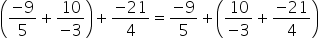Hint:

## The correct answer is: verified.

### To verifyExpress the rational numbers with positive denominator by multiplying with -1.Here,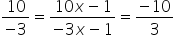Consider the LHS part, we have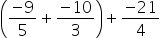…(i)Determine the LCM of the denominators of rational numbers inside the brackets.LCM of 5 and 3 = 15On multiplying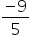with 3, we get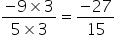On multiplying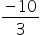with 5, we get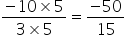On adding, we get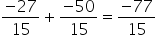On substituting this in (i), we get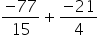Determine the LCM of the denominators of rational numbers.LCM of 15 and 4 = 60On multiplying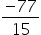with 4, we get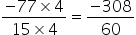On multiplying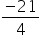with 15, we get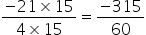On adding, we get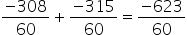Thus we have LHS to be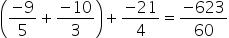Consider the RHS part, we have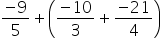…(ii)Determine the LCM of the denominators of rational numbers inside the brackets.LCM of 3 and 4 = 12On multiplyingwith 4, we get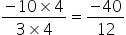On multiplyingwith 3, we get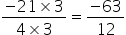On adding, we get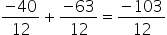On substituting this in (ii), we get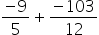Determine the LCM of the denominators of rational numbers.LCM of 5 and 12 = 60On multiplyingwith 12, we get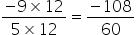On multiplying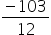with 5, we get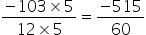On adding, we get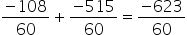Thus we have RHS to be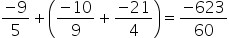So we get LHS = RHS =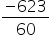Hence verified.#### With Turito Foundation.#### Get an Expert Advice From Turito.